International
Tables for
Crystallography
Volume D
Physical properties of crystals
Edited by A. Authier

International Tables for Crystallography (2006). Vol. D, ch. 2.4, p. 330

## Section 2.4.4.1. Kinematics

R. Vachera* and E. Courtensa

aLaboratoire des Verres, Université Montpellier 2, Case 069, Place Eugène Bataillon, 34095 Montpellier CEDEX, France
Correspondence e-mail:  rene.vacher@ldv.univ-montp2.fr

#### 2.4.4.1. Kinematics

| top | pdf |

Brillouin scattering occurs when an incident photon at frequency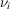interacts with the crystal to either produce or absorb an acoustic phonon at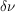, while a scattered photon at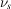is simultaneously emitted. Conservation of energy gives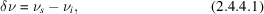where positivecorresponds to the anti-Stokes process. Conservation of momentum can be written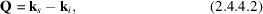where Q is the wavevector of the emitted phonon, and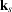,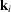are those of the scattered and incident photons, respectively. One can define unit vectors q in the direction of the wavevectors k by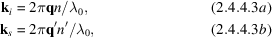where n and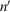are the appropriate refractive indices, andis the vacuum wavelength of the radiation. Equation (2.4.4.3b)assumes that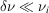so thatis not appreciably changed in the scattering. The incident and scattered waves have unit polarization vectorsand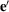, respectively, and corresponding indices n and. The polarization vectors are the principal directions of vibration derived from the sections of the ellipsoid of indices by planes perpendicular toand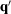, respectively. We assume that the electric vector of the light field Eopt is parallel to the displacement Dopt. This is exactly true for many cases listed in the tables below. In the other cases (such as skew directions in the orthorhombic group) this assumes that the birefringence is sufficiently small for the effect of the angle between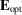and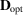to be negligible. A full treatment, including this effect, has been given by Nelson et al. (1972).

After substituting (2.4.4.3)in (2.4.4.2), the unit vector in the direction of the phonon wavevector is given by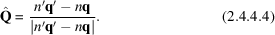The Brillouin shiftis related to the phonon velocity V by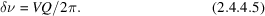Since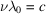, from (2.4.4.5)and (2.4.4.3), (2.4.4.4)one finds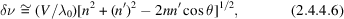whereis the angle betweenand.

### References

Nelson, D. F., Lazay, P. D. & Lax, M. (1972). Brillouin scattering in anisotropic media: calcite. Phys. Rev. B, 6, 3109–3120.Google Scholar